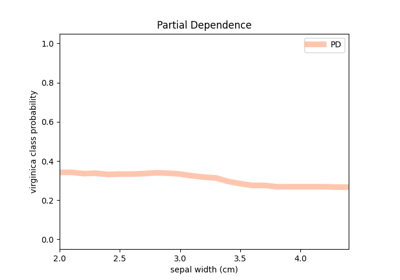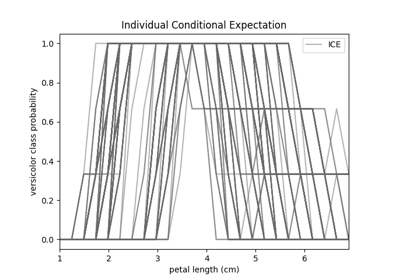# fatf.vis.feature_influence.plot_partial_dependence¶

fatf.vis.feature_influence.plot_partial_dependence(pd_array: numpy.ndarray, feature_linespace: numpy.ndarray, class_index: int, feature_name: Optional[str] = None, class_name: Optional[str] = None, plot_axis: Optional[matplotlib.axes._axes.Axes] = None) → Tuple[Optional[matplotlib.figure.Figure], matplotlib.axes._axes.Axes][source]

Plots Partial Dependence for a selected class.

This function raises the same exceptions and errors as the fatf.vis.feature_influence.plot_individual_conditional_expectation function. Please consult its documentation for the exact list or errors and exceptions and their description.

Parameters
pd_arraynumpy.ndarray

An array of (n_steps, n_classes) shape with Partial Dependence calculation results for every target class for the desired spectrum of the selected feature. This should be the output of the fatf.transparency.models.feature_influence.partial_dependence or fatf.transparency.models.feature_influence.partial_dependence_ice function.

feature_linespacenumpy.ndarray

A one-dimensional array – (steps_number, ) – with the values for which the selected feature was sampled when the dataset was evaluated for a predictive model. This should be the output of the fatf.transparency.models.feature_influence.individual_conditional_expectation function.

class_indexinteger

The index of the class for which PD will be plotted, taken from the original dataset.

feature_namestring, optional (default=None)

The name of the feature for which PD was originally calculated.

class_namestring, optional (default=None)

The name of the class that class_index parameter points to. If None, the class name will be the same as the class index.

plot_axismatplotlib.pyplot.Axes, optional (default=None)

A matplotlib axes on which the PD will be plotted. This is useful if one wants to overlay PD onto an ICE plot. If None, a new axes will be created.

Returns
plot_figureUnion[matplotlib.pyplot.Figure, None]

A matplotlib figure that holds the plot_axis axis. This parameter is None when the user passed in plot_axis attribute, otherwise, when a blank plot is created, this is a figure object holding the plot axis (plot_axis).

plot_axismatplotlib.pyplot.Axes

A matplotlib axes with the PD plot.

## Examples using fatf.vis.feature_influence.plot_partial_dependence¶